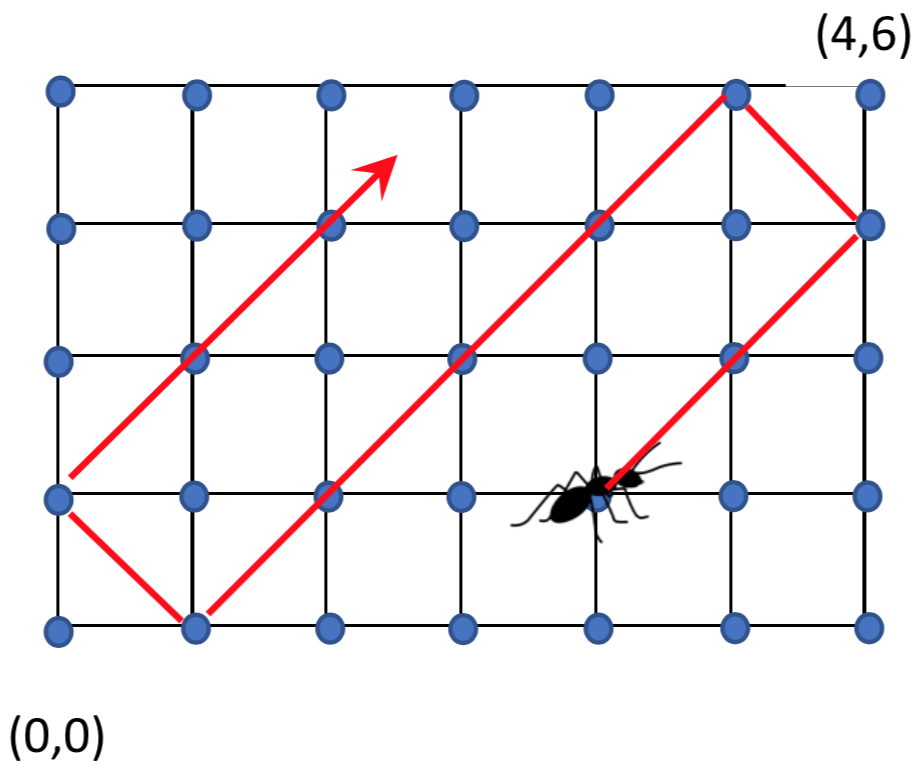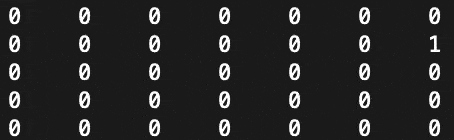# Problem

There is a two-dimensional n by m grid space. This grid is (0,0) lower left and (n, m) upper right as shown below:There is an ant on the coordinates (i, j) in this space. (i=1 and j=4 in the example). The ant begins to move at a constant speed in the right upper 45 ° direction. The ant that first started from (i, j) moves to (i + 1, j + 1) after one hour. However, if hit the interface, it will move to the other direction at the same speed.

Make a program that calculates the position of ant after H hours.

Visual Aid:Input & Output Format

T = number of test case

Input

T

N M I J H

N M I J H

Output

X Y

X Y

Example

Input

2

4 6 1 4 8

5 7 3 5 4

Output

1 0

3 5

# Solution

``````import java.util.Scanner;

public class AntMaze {

private static void print(int[][] map) {
for (int[] a : map) {
for (int b : a) {
System.out.print(b + "\t");
}
System.out.println();
}
System.out.println();
}

public static void main(String[] args) {
String in = "2\n" +
"4 6 1 4 8\n" +
"5 7 3 5 4";

Scanner s = new Scanner(in);

int T = s.nextInt();

for (int t = 0; t < T; t++) {
int N = s.nextInt() + 1;
int M = s.nextInt() + 1;
int I = N - s.nextInt() - 1;
int J = s.nextInt();
int H = s.nextInt();

int[][] map = new int[N][M];

map[I][J] = 1;

int dir = 0;

for (int h = 0; h < H; h++) {
if (I == 0 && J + 1 == M) {
dir = 2;
}
else if (I == 0 && J == 0) {
dir = 3;
}
else if (I + 1 == N && J + 1 == M) {
dir = 1;
}
else if (I + 1 == N && J == 0) {
dir = 0;
}
else if (J + 1 == M) {
if (dir == 0) {
dir = 1;
} else if (dir == 3) {
dir = 2;
}
}
else if (J == 0) {
if (dir == 1) {
dir = 0;
} else if (dir == 2) {
dir = 3;
}
}
else if (I == 0) {
if (dir == 1) {
dir = 2;
} else if (dir == 0) {
dir = 3;
}
}
// check if hit bottom wall
else if (I + 1 == N) {
if (dir == 2) {
dir = 1;
} else if (dir == 3) {
dir = 0;
}
}
map[I][J] = 0;

if (dir == 0) {
I--;
J++;
} else if (dir == 1) {
I--;
J--;
} else if (dir == 2) {
I++;
J--;
} else if (dir == 3) {
I++;
J++;
}

map[I][J] = 1;
print(map);
}

System.out.println((N - I - 1) + " " + J);
}
}
}
``````